# 14-1100/Homework Assignment 4

Jump to: navigation, search

This assignment is due at class time on Thursday, November 20, 2011.

### Solve the following questions

Problem 1. (Klein's 1983 course)

1. Show that the ideal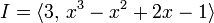$I=\langle 3,\, x^3-x^2+2x-1\rangle$ inside the ring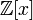${\mathbb Z}[x]$ is not principal.
2. Is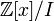${\mathbb Z}[x]/I$ a domain?

Problem 2. Prove that a ring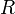$R$ is a PID iff it is a UFD in which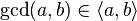$\gcd(a,b)\in\langle a, b\rangle$ for every non-zero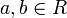$a,b\in R$.

Problem 3. (Lang) Show that the ring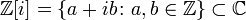${\mathbb Z}[i]=\{a+ib\colon a,b\in{\mathbb Z}\}\subset{\mathbb C}$ is a PID and hence a UFD. What are the units of that ring?

Problem 4. (Dummit and Foote) In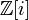${\mathbb Z}[i]$, find the greatest common divisor of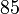$85$ and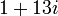$1+13i$, and express it as a linear combination of these two elements.

Problem 5. (Klein's 1983 course) Show that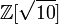${\mathbb Z}[\sqrt{10}]$ is not a UFD.

Problem 6. (Hard!) Show that the quotient ring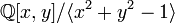${\mathbb Q}[x,y]/\langle x^2+y^2-1\rangle$ is not a UFD.